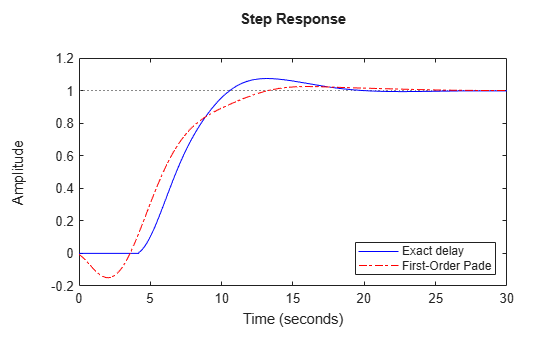## Time-Delay Approximation in Continuous-Time Closed-Loop Model

This example shows how to approximate delays in a continuous-time closed-loop system with internal delays, using `pade`.

Padé approximation is helpful when using analysis or design tools that do not support time delays.

1. Create sample continuous-time closed-loop system with an internal delay.Construct a model `Tcl` of the closed-loop transfer function from `r` to `y`.

```s = tf('s'); G = (s+1)/(s^2+.68*s+1)*exp(-4.2*s); C = pid(0.06,0.15,0.006); Tcl = feedback(G*C,1);```

Examine the internal delay of `Tcl`.

`Tcl.InternalDelay`
```ans = 4.2000 ```
2. Compute the first-order Padé approximation of `Tcl`.

`Tnd1 = pade(Tcl,1);`

`Tnd1` is a state-space (`ss`) model with no delays.

3. Compare the frequency response of the original and approximate models.

```h = bodeoptions; h.PhaseMatching = 'on'; bodeplot(Tcl,'-b',Tnd1,'-.r',{.1,10},h); legend('Exact delay','First-Order Pade','Location','SouthWest');```The magnitude and phase approximation errors are significant beyond 1 rad/s.

4. Compare the time domain response of `Tcl` and `Tnd1` using `stepplot`.

```stepplot(Tcl,'-b',Tnd1,'-.r'); legend('Exact delay','First-Order Pade','Location','SouthEast');```Using the Padé approximation introduces a nonminimum phase artifact (“wrong way” effect) in the initial transient response.

5. Increase the Padé approximation order to see if this will extend the frequency with good phase and magnitude approximation.

`Tnd3 = pade(Tcl,3);`
6. Observe the behavior of the third-order Padé approximation of `Tcl`. Compare the frequency response of `Tcl` and `Tnd3`.

```bodeplot(Tcl,'-b',Tnd3,'-.r',Tnd1,'--k',{.1,10},h); legend('Exact delay','Third-Order Pade','First-Order Pade',... 'Location','SouthWest');```The magnitude and phase approximation errors are reduced when a third-order Padé approximation is used.

Increasing the Padé approximation order extends the frequency band where the approximation is good. However, too high an approximation order may result in numerical issues and possibly unstable poles. Therefore, avoid Padé approximations with order N>10.